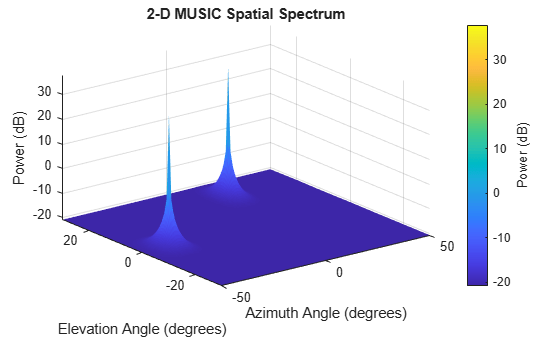# plotSpectrum

System object: phased.MUSICEstimator2D
Package: phased

Plot 2-D MUSIC spectrum

## Syntax

```plotSpectrum(estimator) output_args = plotSpectrum(estimator,Name,Value) lh = plotSpectrum(___) ```

## Description

`plotSpectrum(estimator)` plots the 2-D MUSIC spatial spectrum computed by the most recent `step` method execution for the `phased.MUSICEstimator2D`, `estimator`.

`output_args = plotSpectrum(estimator,Name,Value)` plots the 2-D MUSIC spatial spectrum with additional options specified by one or more `Name,Value` pair arguments.

`lh = plotSpectrum(___)` returns the line handle to the figure.

## Input Arguments

expand all

2-D MUSIC estimator, specified as a `phased.MUSICEstimator2D` System object.

### Name-Value Arguments

Specify optional pairs of arguments as `Name1=Value1,...,NameN=ValueN`, where `Name` is the argument name and `Value` is the corresponding value. Name-value arguments must appear after other arguments, but the order of the pairs does not matter.

Before R2021a, use commas to separate each name and value, and enclose `Name` in quotes.

Units used for plotting, specified as the comma-separated pair consisting of `'Unit'` and `'db'`, `'mag'`, or `'pow'`.

Data Types: `char`

Plot a normalized spectrum, specified as the comma-separated pair consisting of `'NormalizedResponse'` and `false` or `true`. Normalization sets the magnitude of the largest spectrum value to one.

Example: `true`

Data Types: `char`

Title of plot, specified as a comma-separated pair consisting of `'Title'` and a character vector.

Example: `true`

Data Types: `char`

## Output Arguments

expand all

Line handle of plot.

## Examples

expand all

Assume that two sinusoidal waves of frequencies 450 Hz and 600 Hz strike a URA from two different directions. Signals arrive from -37° azimuth, 0° elevation and 17° azimuth, 20° elevation. Use 2-D MUSIC to estimate the directions of arrival of the two signals. The array operating frequency is 150 MHz and the signal sampling frequency is 8 kHz.

```f1 = 450.0; f2 = 600.0; doa1 = [-37;0]; doa2 = [17;20]; fc = 150e6; c = physconst('LightSpeed'); lam = c/fc; fs = 8000;```

Create the URA with default isotropic elements. Set the frequency response range of the elements.

```array = phased.URA('Size',[11 11],'ElementSpacing',[lam/2 lam/2]); array.Element.FrequencyRange = [50.0e6 500.0e6];```

Create the two signals and add random noise.

```t = (0:1/fs:1).'; x1 = cos(2*pi*t*f1); x2 = cos(2*pi*t*f2); x = collectPlaneWave(array,[x1 x2],[doa1,doa2],fc); noise = 0.1*(randn(size(x))+1i*randn(size(x)));```

Create and execute the 2-D MUSIC estimator to find the directions of arrival.

```estimator = phased.MUSICEstimator2D('SensorArray',array,... 'OperatingFrequency',fc,... 'NumSignalsSource','Property',... 'DOAOutputPort',true,'NumSignals',2,... 'AzimuthScanAngles',-50:.5:50,... 'ElevationScanAngles',-30:.5:30); [~,doas] = estimator(x + noise)```
```doas = 2×2 -37 17 0 20 ```

The estimated DOAs exactly match the true DOAs.

Plot the 2-D spatial spectrum.

`plotSpectrum(estimator);`## Version History

Introduced in R2016b